search
Search
Publish
Guest 0reps
Thanks for the thanks!
close
chevron_left Linear Regression
Cancel
Post
account_circle
Profile
exit_to_app
Sign out
search
keyboard_voice
close
Searching Tips
Search for a recipe: "Creating a table in MySQL"
Search for an API documentation: "@append"
Search for code: "!dataframe"
Apply a tag filter: "#python"
Useful Shortcuts
/ to open search panel
Esc to close search panel
to navigate between search results
d to clear all current filters
Enter to expand content previewDoc SearchCode Search BetaSORRY NOTHING FOUND!
mic
Start speaking...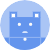Voice search is only supported in Safari and Chrome.
Shrink
Navigate to
A
A
share
thumb_up_alt
bookmark
arrow_backShareTwitterFacebook
chevron_left Linear Regression
thumb_up
1
thumb_down
0
chat_bubble_outline
0
auto_stories new
settings

# Introduction to Linear Regression

Machine Learning
chevron_right
Linear Regression
schedule Mar 10, 2022
Last updated
local_offer
Tags

The objective of linear regression is to draw a line of best fit that can then be used for predictions and inferences. Out of millions of lines that can be drawn to model a given a set of points, how do we know which one is better than the others?

# Cost functions

Consider the following two lines: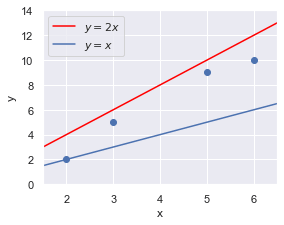Intuitively, we can tell that the red line fits our data-points better than the blue line since the red line is relatively closer to the data-points.

By convention in machine learning, the performance of a model is based on how inaccurate it is, rather than how accurate it is. The two opposing interpretations are actually identical since, needless to say, describing a model as inaccurate means that the model is not at all accurate. As we shall see in a bit, quantifying how "bad" a model is, is much easier than quantifying how "good" a model is.

In this context of linear regression, this means that we want to measure how far off a model's fitted points are to the actual data points. A line that is only off by a tiny amount obviously implies that the line does an excellent job at modelling the data points.

Now, instead of talking about the performance of a model in abstract terms, we want to come up with a mathematical expression to quantify this notion of how off the model is. In machine learning, this expression is known as the cost function. Cost functions play a central role in machine learning, and they are essentially mathematical expressions that capture how inaccurate a model is. A high value for the cost function means that the model is performing badly, while a low value means that the model is performing well.

# Formulating the cost function

In the previous section, we discussed about how we want to derive the cost function, that is a mathematical expression to quantify how off the model is, for linear regression.

Fortunately, this turns out to be extremely easy - all we have to do is to compute the distances between our data-points and the corresponding points on the fitted line, and then sum them up. Using our previous example, this means that we just need to compute the sum of the red dashed lines as well as the sum of the blue dashed lines: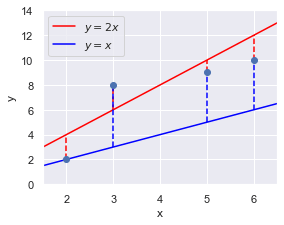Mathematically, this translates to computing the following:

$$J=\frac{1}{m}\sum^m_{i=1}\left(y_i-\hat{y}_i\right)^2$$

Where,

• $J$ is the cost function, which represents the quantity we want to minimise

• $m$ is the number of data-points ($4$ in this case)

• $y_i$ is the $y$ value of the $i$-th data-point (e.g. $y_2=8$)

• $\hat{y}_i$ is the fitted $y$ value of the $i$-th data-point (e.g. $\hat{y}_2=6$ for the red fitted line)

Formulating the cost function is only half the battle won - we now need to derive the parameters of the model that would minimise the cost function.

NOTE

The cost function for linear regression is often referred to as the sum of squares of error (SSE) in statistics. Different names - same idea.

## Purpose of squaring the difference

Notice how we have the term $(y_i−\hat{y}_i)^2$​​​ in the cost function, which you might find strange since ​$y_i−\hat{y}_i$​ already captures the accuracy of our model.

The reason for this is that whether or not our data-points are above or below the fitted line should not matter - we are only concerned about how off we are. Without the square, we would end up having positive and negative differences:

• when the estimated value ($\hat{y}_i$) is smaller than the actual value ($y_i$), then $y_i-\hat{y}_i$ will be positive.

• when the estimated value is larger than the actual value, then $y_i−\hat{y}_i$ will be negative.

Since we need to compute the sum of these differences, the positives and negatives cancel each other out, thereby erroneously reducing the the total error.

Just to show this graphically, suppose we have the following scenario: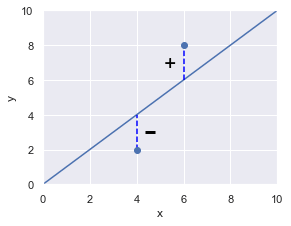If we did not include the square term there, then the error terms would simply cancel each other out, and hence the cost function will be evaluated as 0 - a perfect fit! Clearly, this isn't correct since the line does not go through all the points. To avoid traps like this, we must include the square there.

## Squaring versus taking absolute value

Now, you maybe wondering why we take the square instead of taking the absolute value. Indeed, taking the absolute value (i.e. $|y-\hat{y}_i|$) does solve our problem here as well since all the differences will become positive. In fact, you might argue that this provides a better assessment of how inaccurate the model is since taking the square increases the cost function. You are right - but absolute values don't work well with derivatives, which is problematic since we will be taking the derivative of the cost function later on.

Moreover, it does not matter that much that the inaccuracy will be more bloated when we take the square. The reason is two-fold:

• we are typically concerned with finding the parameters that minimise the inaccuracy, that is, the final value of the cost function is less important.

• we can still objectively compare the performance of two models as long as we use the exact same cost function, so the additional bloat caused by the square term matters not.

# Computing the cost function

Now that we've covered why the cost function is written the way that it is, we'll run through a quick example of actually computing the cost function. Recall that our example was as follows:As a refresher, here's the cost function again:

$$J=\frac{1}{m}\sum^m_{i=1}\left(y_i-\hat{y}_i\right)^2$$

To compute the respective cost for the red and blue lines:

\begin{align} \color{#e868a1}{J}&=\frac{1}{4} \left[ (2\cdot2-2)^2+(2\cdot 3-6)^2+(2\cdot5-8)^2+(2\cdot 6-12)^2\right]=3.25 \\ \color{#4fc3f7}{J}&=\frac{1}{4} \left[ (2-2)^2+(2-6)^2+(2-8)^2+(2-12)^2\right]=14.25 \end{align}

We see that $\color{#e868a1}{J}\;\color{#d3d4d6}{<}\;\color{#4fc3f7}{J}$, which confirms our intuition that the red line fits the data-points more accurately. Remember, the cost function represents how off a model is, so the lower the cost function, the better the model is.

# Finding the line of best fit

In the previous section, we compared the performance of two models by computing their cost function. We are now ready to tackle the more interesting challenge of actually finding the line of best fit, which entails deriving the parameters of the line.

In our example, we are only interested in finding the line of best fit that goes through the origin, so the parameter that we want to find is just the slope. A line that passes through the origin will always be of the following form:

$$\hat{y}=\theta x$$

Here, $\theta$ represents the slope of the line, and it is the parameter that we want to optimise.

Just a reminder, the form of the cost function we dealt with previously was as follows:

$$J=\frac{1}{2m}\sum^m_{i=1}\left(y_i-\hat{y}_i\right)^2$$

We now want to rewrite this cost function for our specific scenario. We've established that the line we want to fit is $\hat{y}=\theta x$, so substituting this into our cost function gives:

$$J=\frac{1}{2m}\sum^m_{i=1}\left(y_i-\hat{y}_i\right)^2 \qquad \Leftrightarrow \qquad J(\theta)=\frac{1}{2m}\sum^m_{i=1}\left(y_i-\theta{x_i} \right)^2$$

Notice how the $J$ has been rewritten as $J(\theta)$, which is to say that our cost function is dependent on $\theta$. Remember, our data-points $(x_i,y_i)$ are fixed, so it should be clear that the cost function is only dependent on $\theta$. To make this clear, let's actually determine the explicit form of $J(\theta)$ by substituting in our data-points:

$$J(\theta)=\frac{1}{6}\left[(2-2\theta)^2 + (8-3\theta)^2+(9-5\theta)^2+(10-6\theta)^2 \right]$$

This is exactly what we've done in the previous section, but the only difference is that, instead of computing the cost function of a defined line (e.g. $y=2x$), we are now computing the cost function of a general line (i.e. $y=\theta x$).

Simplifying this gives us the following:

$$J(\theta)=\frac{1}{6}\left(74\theta^2-266\theta+249\right)$$

We see that our $J(\theta)$ is nothing more than a parabola, which visually looks like the following: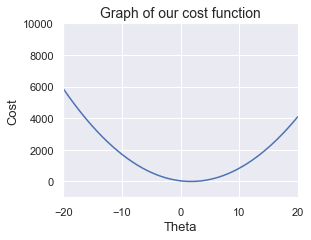We can see that the cost is minimised when $\theta\approx2$. Instead of eyeballing like so, we will now compute the actual $\theta$ value that minimises the cost function.

# Minimising the cost function

There are two common ways to minimise the cost function.

The first way is the analytical approach where we use old-school calculus - take the first derivative and equate it to zero. This approach gives you exact answers, but for complex models, computing the derivative would require too much time.

The second way is the numerical approach where we rely on algorithms designed to find the optimal solution. Although this approach may not always return the optimal solution, it can handle complex models far better than the analytical approach.

In statistics, we normally use the analytical approach, whereas in machine learning, the numerical approach is more commonplace. Since this is a tutorial about linear regression in the context of machine learning, we will briefly introduce the numerical approach. If you'd like to learn about the analytical approach, click here for a tutorial about statistical linear regression.

Gradient descent is a general algorithm that aims to find values that minimise a particular function. Our ultimate goal is to apply gradient descent to compute the parameter, $\theta$, that minimises the cost function.

We won't explain how gradient descent works here as we've already done so in our tutorial here that is specifically about gradient descent, so please check that out first and come back here.

* * *

The update rule of gradient descent for our cost function $J(\theta)$ is as follows:

$$\theta$$

Here, we shall use the following parameters for gradient descent:

 starting theta = 15learning rate = 0.01iterations = 1000 

When we apply gradient descent, we end up with the following result: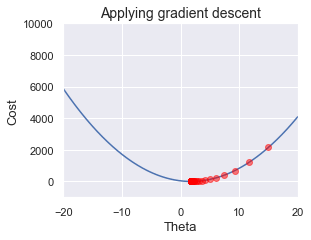As we can see, we start from $\theta=15$ and we are making our way downwards to the minimum of the cost function at each iteration of the gradient descent. The final result of gradient descent is as follows:

 theta = 1.7972972972976708cost function = 1.6599099099099086 

Just as a comparison, recall that the cost function of the lines $\color{#e868a1}y=2x$ and $\color{#4fc3f7}y=x$ were as follows:

\begin{align} \color{#e868a1}{J}&=3.25 \\ \color{#4fc3f7}{J}&=14.25 \end{align}

Our cost function when $\theta\approx1.8$ is around $1.66$, which means that our model has a lower cost function than either of these lines. Again, this is to be expected since the gradient descent returns a value for $\theta$ that minimises the cost function.

So the optimal $\theta$ is around $1.8$, which means that the line of best fit is as follows:

$$y=\theta x \qquad \Leftrightarrow \qquad \color{#71bf5d}y=1.8 x$$

Great, let's visualise line to see just how well it fits the actual data points: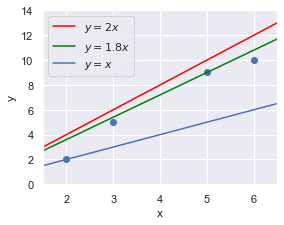We can visually see that the our model (green line) performs a lot better than the blue line, and slightly better than the red line. To reiterate, we know for a fact that $y=1.8x$ is the best line - no other line can top its accuracy.